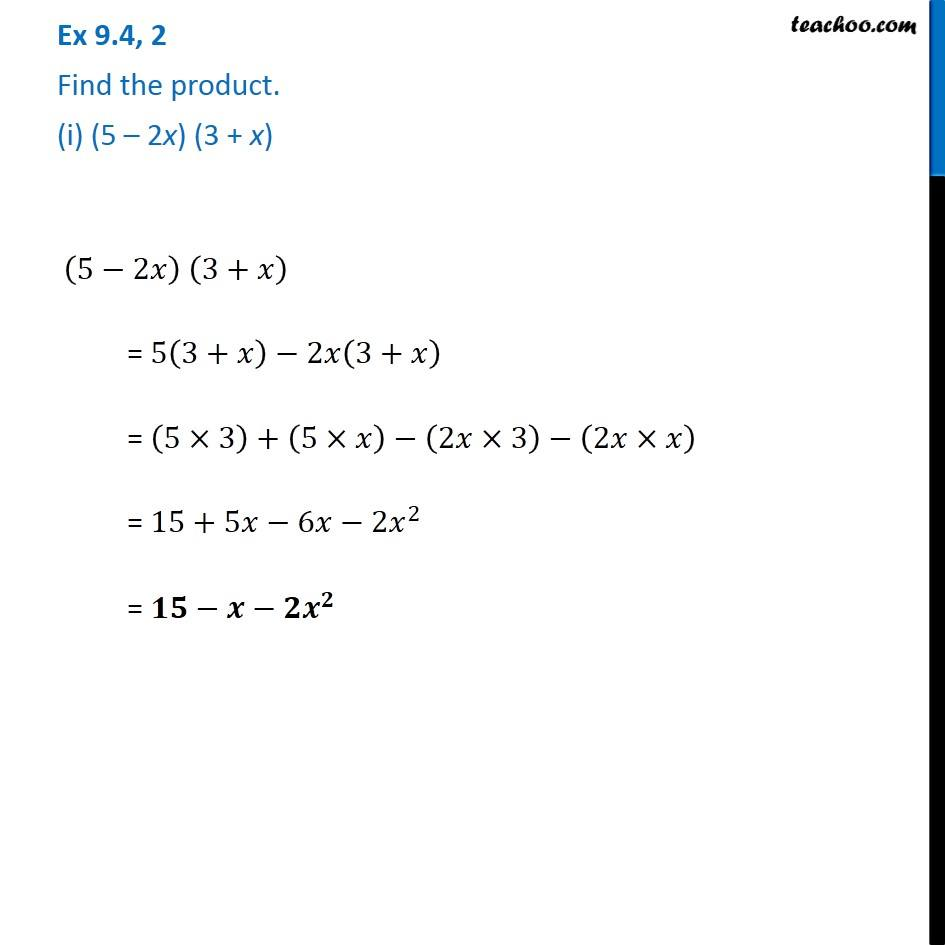Subscribe to our Youtube Channel - https://you.tube/teachoo

1. Chapter 9 Class 8 Algebraic Expressions and Identities
2. Concept wise
3. Multiplication of Polynoimals by Polynomials

Transcript

Ex 9.4, 2 Find the product. (i) (5 – 2x) (3 + x) (5−2𝑥) (3+𝑥) = 5(3+𝑥)−2𝑥(3+𝑥) = (5×3)+(5×𝑥)−(2𝑥×3)−(2𝑥×𝑥) = 15+5𝑥−6𝑥−2𝑥^2 = 𝟏𝟓−𝒙−𝟐𝒙^𝟐

Multiplication of Polynoimals by Polynomials